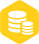# 标准频带FIR滤波器设计

1.1w人已加入

### 描述

FIR（Finite Impulse Response，有限脉冲响应）滤波器是一种数字滤波器，其输出信号仅由输入信号和滤波器的冲激响应决定。FIR滤波器的名称源于其冲激响应是一个有限长度的序列。

FIR滤波器的特点是具有稳定性、线性相位特性和精确控制频率响应的能力。它通过将输入信号与滤波器的冲激响应进行卷积运算来实现信号的滤波效果。FIR滤波器在实际应用中广泛用于滤除噪声、提取信号特征、平滑数据等。

FIR滤波器的设计涉及选择滤波器的阶数（即冲激响应的长度）和确定滤波器的系数。常见的FIR滤波器设计方法包括窗函数法、频率采样法、最小最大法等。设计滤波器的目标是在满足特定频率响应需求的同时，尽可能减小滤波器的阶数和降低误差。

FIR滤波器具有一些优点，例如相对较简单的实现、稳定性和可控的频率响应。然而，较高阶数的FIR滤波器可能需要更多的计算资源，并且在实时应用中可能引入一定的延迟。

FIR（Finite Impulse Response，有限脉冲响应）滤波器是一种数字滤波器，其原理可以从时域和频域两个方面进行解释。

2.1时域原理

FIR滤波器的输出是通过将输入信号与滤波器的冲激响应进行卷积得到的。冲激响应是滤波器对单位脉冲输入信号的响应，它描述了滤波器在时域上的特性。FIR滤波器的冲激响应是一个有限长度的序列，因此称为有限脉冲响应滤波器。

FIR滤波器的输出可以用以下公式表示：

``````y[n] = b*x[n] + b*x[n-1] + b*x[n-2] + ... + b[N]*x[n-N]
``````

2.2频域原理

FIR滤波器的频率响应可以用以下公式表示：

``````H(f) = be^(-j2pif0) + be^(-j2pif1) + ... + b[N]e^(-j2pif*N)
``````

FIR滤波器的频率响应可以通过选择合适的冲激响应系数来控制，从而实现对不同频率信号的滤波效果。不同的冲激响应系数会导致不同的频率响应特性，例如低通、高通、中通或带阻等滤波器类型。

fir1/firwin使用最小二乘逼近计算滤波器系数，然后通过加窗对脉冲响应进行平滑处理。标准频带FIR滤波器是一种常见的设计方法，用于满足特定的频率响应需求。该设计方法旨在设计一个FIR滤波器，使其在指定的频率范围内具有预期的增益或衰减特性。下面是标准频带FIR滤波器的设计步骤：

（1）确定设计规格：

（2）选择窗函数：

（3）确定滤波器的长度：

（4）设计滤波器：

（5）可选的优化步骤：

（6）应用滤波器：

Python中的SciPy库提供了fir1/firwin函数，可以方便地进行标准频带FIR滤波器的设计。

firwin函数：

``````firwin(numtaps, cutoff, width=None, window='hamming', pass_zero=True, scale=True, fs=2.0)
``````

numtaps ：滤波器的长度（阶数）。

cutoff ：截止频率或截止频率数组。对于低通和高通滤波器，可以是一个标量值；对于带通和带阻滤波器，可以是包含两个截止频率的列表或数组。

width ：仅在带通和带阻滤波器中使用，表示过渡带（transition band）的宽度。

window ：窗函数的类型，默认为汉明窗（'hamming'）。

pass_zero ：确定滤波器是否通过零频率点。对于低通和带通滤波器，为True表示通过零频率；对于高通和带阻滤波器，为False表示不通过零频率。

scale ：指定是否对滤波器系数进行缩放。

fs ：采样频率。

fir1函数：

``````fir1(numtaps, cutoff, window='hamming', pass_zero=True, scale=True, fs=2.0)
``````

fir1函数返回滤波器的冲激响应系数，可以使用这些系数应用于输入信号。

``````from scipy.signal import firwin, lfilter
import numpy as np
import matplotlib.pyplot as plt

# 设计规格
numtaps = 101  # 滤波器长度
cutoff = 0.4  # 截止频率
fs = 1.0  # 采样频率

# 使用firwin函数设计低通FIR滤波器
filter_coeff = firwin(numtaps, cutoff, fs=fs)

# 打印滤波器系数
print("Filter Coefficients:", filter_coeff)

# 应用滤波器
input_signal = np.random.randn(1000)  # 输入信号为随机噪声
output_signal = lfilter(filter_coeff, 1, input_signal)

# 绘制输入信号和输出信号的时域波形
time = np.arange(0, len(input_signal)) / fs
plt.figure()
plt.plot(time, input_signal, label='Input Signal')
plt.plot(time, output_signal, label='Filtered Signal')
plt.xlabel('Time')
plt.ylabel('Amplitude')
plt.title('Time Domain Waveform')
plt.legend()
plt.grid(True)
plt.show()
``````

``````from scipy.signal import firwin, lfilter
import numpy as np
import matplotlib.pyplot as plt

# 设计规格
numtaps = 101  # 滤波器长度
cutoff = [0.2, 0.6]  # 截止频率范围
fs = 1.0  # 采样频率

# 使用firwin函数设计中通FIR滤波器
filter_coeff = firwin(numtaps, cutoff, fs=fs, pass_zero=False)

# 打印滤波器系数
print("Filter Coefficients:", filter_coeff)

# 应用滤波器
input_signal = np.random.randn(1000)  # 输入信号为随机噪声
output_signal = lfilter(filter_coeff, 1, input_signal)

# 绘制输入信号和输出信号的时域波形
time = np.arange(0, len(input_signal)) / fs
plt.figure(figsize=(10, 4))
plt.plot(time, input_signal, label='Input Signal')
plt.plot(time, output_signal, label='Filtered Signal')
plt.xlabel('Time')
plt.ylabel('Amplitude')
plt.title('Time Domain Waveform')
plt.legend()
plt.grid(True)
plt.show()
``````

firwin函数在设计高通（highpass）和低通（lowpass）FIR滤波器时具有一些差异：

``````from scipy.signal import firwin, lfilter
import numpy as np
import matplotlib.pyplot as plt

# 设计规格
numtaps = 101  # 滤波器长度
cutoff = 0.4  # 截止频率
fs = 1.0  # 采样频率

# 使用firwin函数设计高通FIR滤波器
filter_coeff = firwin(numtaps, cutoff, fs=fs, pass_zero=False)

# 打印滤波器系数
print("Filter Coefficients:", filter_coeff)

# 应用滤波器
input_signal = np.random.randn(1000)  # 输入信号为随机噪声
output_signal = lfilter(filter_coeff, 1, input_signal)

# 绘制输入信号和输出信号的时域波形
time = np.arange(0, len(input_signal)) / fs
plt.figure(figsize=(10, 4))
plt.plot(time, input_signal, label='Input Signal')
plt.plot(time, output_signal, label='Filtered Signal')
plt.xlabel('Time')
plt.ylabel('Amplitude')
plt.title('Time Domain Waveform')
plt.legend()
plt.grid(True)
plt.show()
``````

firwin函数在设计带通（bandpass）和带阻（bandstop）FIR滤波器时具有一些差异：

``````from scipy.signal import firwin, lfilter
import numpy as np
import matplotlib.pyplot as plt

# 设计规格
numtaps = 101  # 滤波器长度
cutoff = [0.4, 0.6]  # 截止频率范围
fs = 1.0  # 采样频率

# 使用firwin函数设计带阻FIR滤波器
filter_coeff = firwin(numtaps, cutoff, fs=fs, pass_zero=True)

# 打印滤波器系数
print("Filter Coefficients:", filter_coeff)

# 应用滤波器
input_signal = np.random.randn(1000)  # 输入信号为随机噪声
output_signal = lfilter(filter_coeff, 1, input_signal)

# 绘制输入信号和输出信号的时域波形
time = np.arange(0, len(input_signal)) / fs
plt.figure(figsize=(10, 4))
plt.plot(time, input_signal, label='Input Signal')
plt.plot(time, output_signal, label='Filtered Signal')
plt.xlabel('Time')
plt.ylabel('Amplitude')
plt.title('Time Domain Waveform')
plt.legend()
plt.grid(True)
plt.show()
``````

Kaiser窗法（Kaiser window method）是一种用于FIR滤波器设计的方法，它利用Kaiser窗函数来估计滤波器的阶数（即滤波器长度）。Kaiser窗函数是一种基于贝塞尔函数的调制函数，具有可调节的参数β，可以控制窗函数的主瓣宽度和副瓣衰减。

Kaiser窗阶估计的步骤如下：

（1）确定设计规格：包括滤波器的截止频率、过渡带宽、最大衰减和窗函数的形状参数β。

（2）计算滤波器的阶数：根据设计规格和窗函数的参数β，使用公式或表格来计算滤波器的阶数。一种常用的计算公式是根据贝塞尔函数的零阶近似计算：

scssCopy codeN = (A - 8) / (2.285 * 2π * Δf) + 1

（3）生成Kaiser窗函数：使用计算得到的阶数N和参数β，生成Kaiser窗函数。Kaiser窗函数可以通过NumPy中的kaiser函数来生成。

（4）设计FIR滤波器：使用firwin函数，将生成的Kaiser窗函数作为窗函数参数，指定其他设计规格（如截止频率、过渡带宽等），生成最终的FIR滤波器系数。

``````from scipy.signal import firwin, kaiser
import numpy as np
import matplotlib.pyplot as plt

# 设计规格
cutoff = 0.2  # 截止频率
transition_width = 0.1  # 过渡带宽
attenuation = 60  # 最大衰减（单位：分贝）
sampling_freq = 1000  # 采样频率

# 计算滤波器的阶数
delta_freq = transition_width * 0.5
N = int((attenuation - 7.95) / (2.285 * 2 * np.pi * delta_freq) + 1)

# 生成Kaiser窗函数
beta = kaiser_beta = np.kaiser_beta(attenuation)
window = kaiser(N, beta)

# 设计FIR滤波器
filter_coeff = firwin(N, cutoff, window=window, fs=sampling_freq)

# 打印滤波器系数
print("Filter Coefficients:", filter_coeff)

# 绘制滤波器的频率响应
freq, response = scipy.signal.freqz(filter_coeff)
magnitude = np.abs(response)
magnitude_db =
``````

fir2/firwin2函数还可用于设计加窗的FIR滤波器，但具有任意形状的分段线性频率响应。这与fir1/firwin不同，后者仅设计具有标准低通、高通、带通和带阻配置的滤波器。

``````scipy.signal.fir2(numtaps, freq, gain, fs=2.0, window='hamming', nyq=None, antisymmetric=False)
``````

numtaps（整数） ：滤波器的长度（阶数），决定了滤波器的频率响应的分辨率和计算复杂度。

freq（数组）： 包含频率点的序列，用于定义滤波器的频率响应。频率范围为0到0.5（归一化频率），其中0.5对应采样频率的一半。

gain（数组）： 与频率点相对应的增益值，用于定义滤波器的目标频率响应。

fs（标量）： 采样频率，用于指定频率的单位。默认为2.0，表示采样频率的一半。如果nyq参数也提供了，则fs参数会被忽略。

window（字符串或数组） 指定窗函数的类型或窗函数自身。可以使用字符串指定标准窗函数，如'hamming'、'hann'、'blackman'等。也可以直接传递一个窗函数数组，长度为numtaps，用于自定义窗函数。

nyq（标量）： 采样频率的一半，用于指定频率的单位。如果未提供，则默认为fs/2.0。

antisymmetric（布尔值）： 指定滤波器是否为反对称滤波器。默认为False，表示滤波器为一般的线性相位滤波器。

h（一维数组）： FIR滤波器的系数。

``````scipy.signal.firwin2(numtaps, freq, gain, fs=2.0, window='hamming', nyq=None)
``````

numtaps（整数）： 滤波器的长度（阶数），决定了滤波器的频率响应的分辨率和计算复杂度。

freq（一维数组）： 频率段边界点的列表或数组，用于定义滤波器的频率响应。频率段边界点应在0到fs/2之间，其中fs为采样频率。

gain（一维数组）： 与频率段相应的增益值的列表或数组，用于定义滤波器的目标频率响应。

fs（标量）： 采样频率，用于指定频率的单位。默认为2.0，表示采样频率的一半。

window（字符串或数组）： 指定窗函数的类型或窗函数自身。可以使用字符串指定标准窗函数，如'hamming'、'hann'、'blackman'等。也可以直接传递一个窗函数数组，长度为numtaps，用于自定义窗函数。

nyq（标量）： 采样频率的一半，用于指定频率的单位。如果未提供，则默认为fs/2.0。

h（一维数组）： FIR滤波器的系数。

``````from scipy.signal import firwin2, freqz
import matplotlib.pyplot as plt

# 定义频率点
freq = [0.0, 0.1, 0.2, 0.3, 0.4, 0.5]
# 定义增益值
gain = [1.0, 1.0, 0.0, 0.0, 1.0, 1.0]

# 设计多频带FIR滤波器
filter_coeff = firwin2(101, freq, gain)

# 打印滤波器系数
print("Filter Coefficients:", filter_coeff)

# 绘制滤波器的频率响应
w, h = freqz(filter_coeff)
plt.plot(w, abs(h))
plt.xlabel('Frequency')
plt.ylabel('Magnitude')
plt.title('Frequency Response')
plt.grid(True)
plt.show()
``````×
20